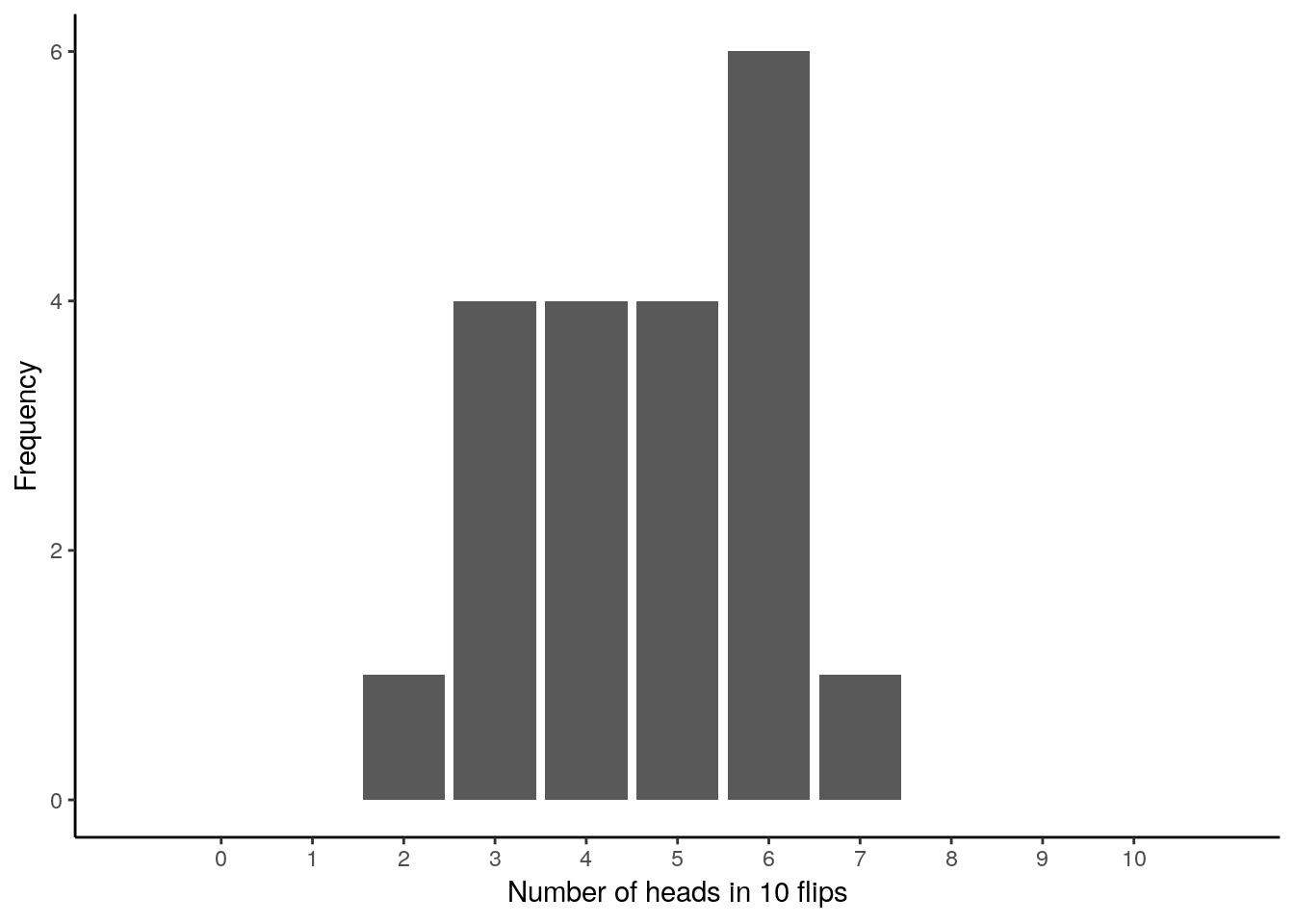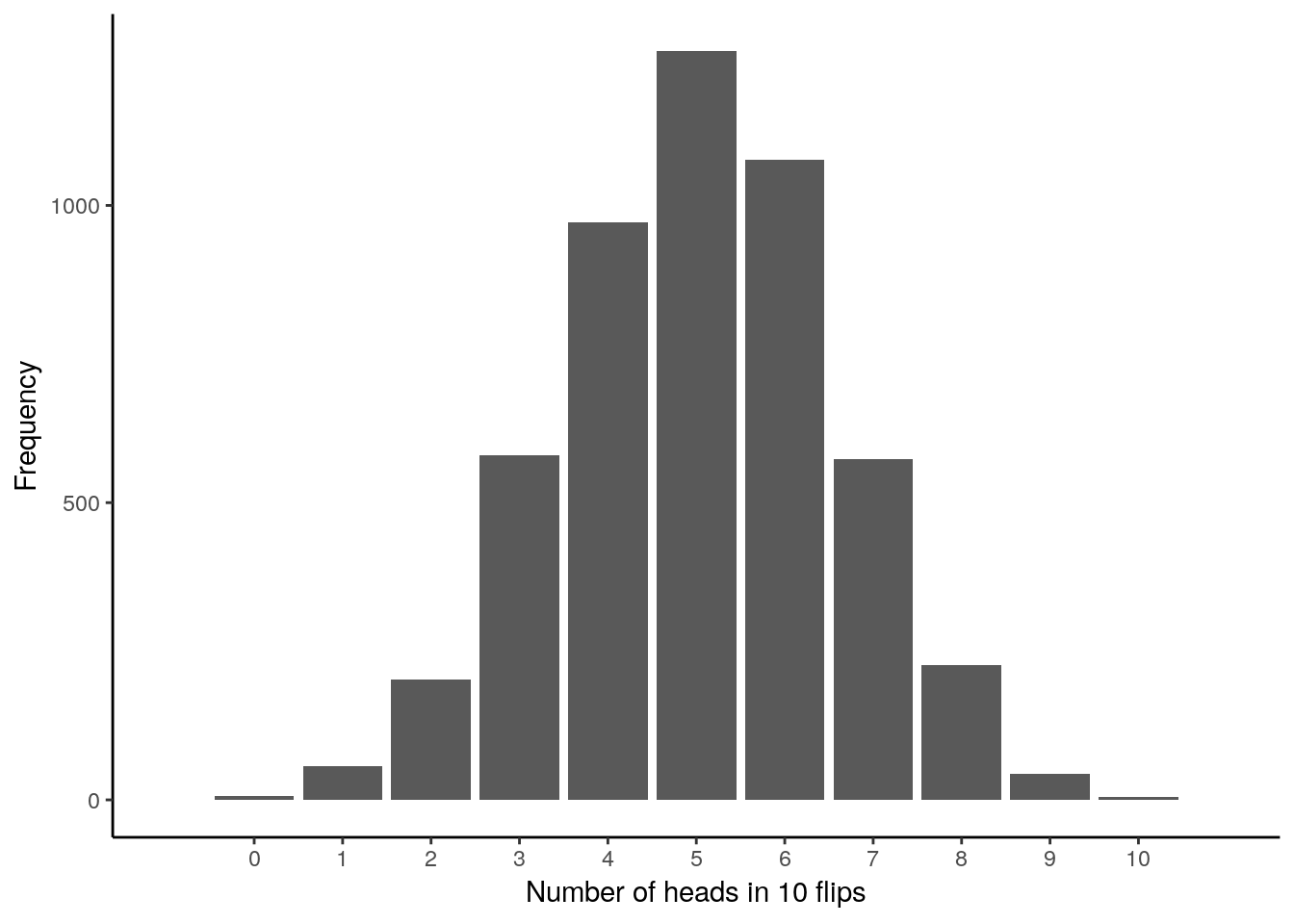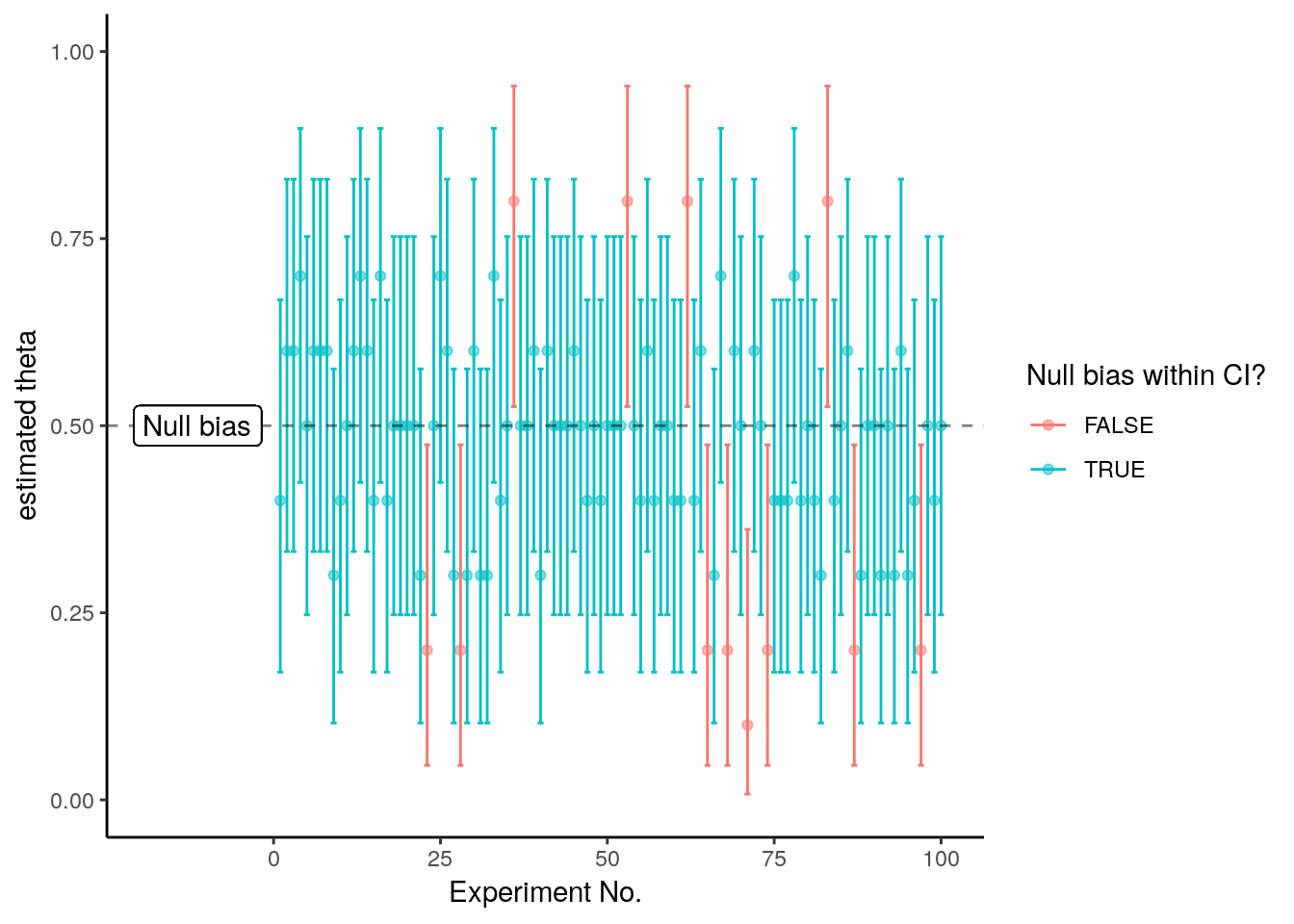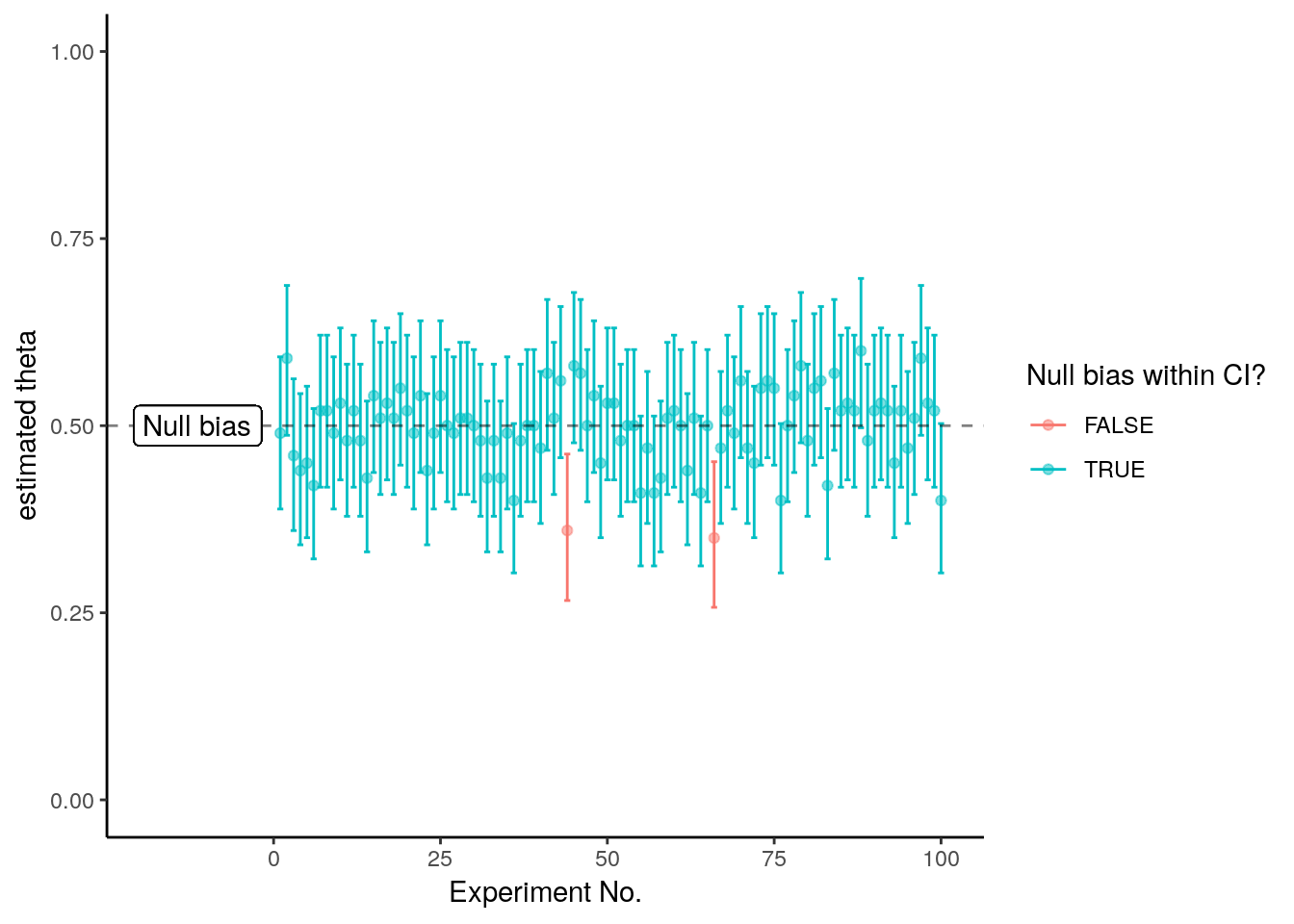# Instructions

• Download and extract this zip file. You will find the R Markdown (.Rmd) file inside.
• Open the .Rmd file in RStudio.
• Fill in the required code and answers.
• ‘Knit’ the document (ctrl/cmd + shift + K in RStudio) to produce a HTML file.

# Required R packages

• rmarkdown (comes with RStudio)
• tidyverse (or ggplot2, purrr, dplyr, tidyr, tibble)
• TeachingDemos
library(tidyverse)
library(TeachingDemos)

# 1. Testing the bias of a coin

This task is all about a coin. Imagine you’re about to make a life changing decision based on a coin flip (don’t do this at home!). First you want to check whether or not the coin is fair. You decide to use your knowledge of experiments and statistics to determine this!

You flip the coin 10 times and count how many times it comes up heads. You count 8 heads. Is the coin biased? Let’s find out!

# # Uncomment and change your lastname
#
# lastname <- "yourlastname"
#
# char2seed(lastname)
# SOLUTION:

lastname <- "bayes"

char2seed(lastname)

## a. Understanding the binomial distribution

The number of heads in a set of coin flips follows the binomial distribution:

$\text{Binom}(K = k ; n, \theta) = \binom{n}{k} \, \theta^{k} \, (1-\theta)^{n-k}$

Here $$\binom{n}{k} = \frac{n!}{k!(n-k)!}$$ (said “n choose k”) is the binomial coefficient. It gives the number of possibilities of drawing an unordered set with $$k$$ elements from a set with a size of $$n$$. $$\theta$$ is the bias of the process (the probability of a single success).

The binomial distribution is built into R. The following commands allow you to interact with it:

• Density:
• dbinom(x, size, prob, log = FALSE)
• Cumulative probability distribution:
• pbinom(q, size, prob, lower.tail = TRUE, log.p = FALSE)
• Quantile:
• qbinom(p, size, prob, lower.tail = TRUE, log.p = FALSE)
• Random generation:
• rbinom(n, size, prob)

You can read about these functions, including the input arguments, by running ?Binomial in the console.

## b. Simulating a fair coin

You realise a reasonable approach would be to investigate how a fair coin would behave. Unfortunately you don’t have a coin that you know is fair (otherwise you’d probably use it for your decision) so you decide to simulate one in R.

Uncomment and fill in the ??s in the code to see the result of flipping a fair coin 10 times. That is, a single experiment of 10 trials.

# # Uncomment and fill in the code
#
# n_exp <- 1
# trials <- ??
# theta <- ??
#
# outcome <- rbinom(n = n_exp, size = trials, prob = theta)
#
# print(outcome)
# SOLUTION:

n_exp <- 1
trials <- 10
theta <- 0.5

outcome <- rbinom(n = n_exp, size = trials, prob = theta)

print(outcome)
##  6

SOLUTION: 6

## c. Multiple experiments

But that was just a single experiment. Being quite an intelligent statistician, you realise that to get a good estimate, you should make more than one simulation. You decide to simulate the experiment 20 times and plot the results.

Fill in the code to do this:

# # Uncomment and fill in the code
#
# n_exp <- ?? # number of experiments
# trials <- ?? # number of trials in an experiment
# theta <- ?? # bias
#
# outcomes <- data_frame(outcomes = rbinom(n_exp, trials, theta))
#
# outcomes %>%
#   ggplot(aes(outcomes)) +
#   geom_bar() +
#   scale_x_continuous(breaks = 0:trials, limits = c(-1, trials + 1)) +
#   xlab("Number of heads in ?? flips") +
#   ylab("Frequency") +
#   theme_classic()
# SOLUTION:

n_exp <- 20 # number of experiments
trials <- 10 # number of trials in an experiment
theta <- 0.5 # bias

outcomes <- data_frame(outcomes = rbinom(n_exp, trials, theta))

outcomes %>%
ggplot(aes(outcomes)) +
geom_bar() +
scale_x_continuous(breaks = 0:trials, limits = c(-1, trials + 1)) +
xlab("Number of heads in 10 flips") +
ylab("Frequency") +
theme_classic()What was the most frequently observed number of heads?

SOLUTION: 6

## d. More experiments

You realise doing only 20 simulations isn’t really enough for a nice graph. So you try doing 5000, because with modern technology, why not?

# # Uncomment and fill in the code
#
# n_exp <- ?? # number of experiments
# trials <- ?? # number of trials in an experiment
# theta <- ?? # bias
#
# outcomes <- data_frame(outcomes = rbinom(n_exp, trials, theta))
#
# outcomes %>%
#   ggplot(aes(outcomes)) +
#   geom_bar() +
#   scale_x_continuous(breaks = 0:trials, limits = c(-1, trials + 1)) +
#   xlab("Number of heads in ?? flips") +
#   ylab("Frequency") +
#   theme_classic()
# SOLUTION:

n_exp <- 5000 # number of experiments
trials <- 10 # number of trials in an experiment
theta <- 0.5 # bias

outcomes <- data_frame(outcomes = rbinom(n_exp, trials, theta))

outcomes %>%
ggplot(aes(outcomes)) +
geom_bar() +
scale_x_continuous(breaks = 0:trials, limits = c(-1, trials + 1)) +
xlab("Number of heads in 10 flips") +
ylab("Frequency") +
theme_classic()## e. Hypotheses

So you have a pretty nice graph. This graph is beginning to approximate the sampling distribution. You see that in some of the simulated experiments, the fair coin showed 8 heads out of 10, but not that frequently.

You realise you need a testable hypothesis about your coin. You remember that in classical statistics you have two hypotheses: the null hypothesis $$H_0$$ and the alternative hypothesis $$H_1$$.

What would these hypotheses be?

SOLUTION: $$H_0$$: The coin is fair ($$\theta = 0.5$$); $$H_1$$: The coin is unfair ($$\theta \neq 0.5$$)

## f. Testing the null-hypothesis with p-values

To test the null hypothesis, you first decide on a false-positive rate (the acceptable rate that this procedure will claim the coin is unfair when it is actually fair). In this case you choose 15%. You then calculate the probability of the observed data, assuming the null hypothesis is true.

You do this by summing up the probability mass for 8, 9 or 10 heads using dbinom(). Because you had no reason to believe the coin was biased in one particular direction (e.g. more biased to heads or more biased to tails) before seeing the data you should also add the mass for 0, 1, or 2 heads. This is then the probability of getting 8 or more heads or 8 or more tails from 10 flips. You compare this value to the false positive rate. This is called a “two-tailed” test.

# # Uncomment and fill in the code
#
# outcomes <- c(??,??,??,??,??,??)
# trials <- ??
# theta <- ??
#
# sum(dbinom(x = outcomes, size = trials, prob = theta))
# SOLUTION:

outcomes <- c(0, 1, 2, 8, 9, 10)
trials <- 10
theta <- 0.5

sum(dbinom(x = outcomes, size = trials, prob = theta))
##  0.109375

What is the probability of the data assuming the null hypothesis is true? Is this higher or lower than your acceptable false positive rate?

SOLUTION: The probability of observing the given proportion (8 out of 10) or more extreme is .109

This value is also called a p-value. Again: A p-value is the long-run probability that the data would come up as observed (or more extreme) if the null hypothesis is true.

Compare your outcome to the number provided by the function binom.test() after inserting the correct information in the following template:

# # Uncomment and fill in the code
#
# binom.test(x = ??, n = ??, p = ??, alternative = "two.sided")
# SOLUTION:

binom.test(x = 8, n = 10, p = 0.5, alternative = "two.sided")
##
##  Exact binomial test
##
## data:  8 and 10
## number of successes = 8, number of trials = 10, p-value = 0.1094
## alternative hypothesis: true probability of success is not equal to 0.5
## 95 percent confidence interval:
##  0.4439045 0.9747893
## sample estimates:
## probability of success
##                    0.8

## g. Confidence intervals

You remember that you can also investigate the null-hypothesis with confidence intervals.

You remember something about confidence intervals from your statistics class. You remember that a 95% confidence interval is the range of values that …

Your memory is hazy, so you decide not to trust it and check Wikipedia instead.

It says: “…[I]f confidence intervals are constructed using a given confidence level from an infinite number of independent sample statistics, the proportion of those intervals that contain the true value of the parameter will be equal to the confidence level.”

So you interpret that to mean that if you calculate a hundred 85% confidence intervals from a hundred different experiments with a fair coin, around 85 of them will cover the value 0.5. Is that intuitive? If it’s not, perhaps making a visualisation will help.

# # Uncomment and fill in the code
#
# n_exp <- ?? # number of simulated experiments
# trials <- ?? # number of coin flips per experiment
# theta <- ?? # null bias
# conf_level <- ?? # confidence level
#
# # simulate the experiments
# outcomes <- rbinom(n = ??,
#                    size = ??,
#                    prob = ??)
#
# # create empty tibble to record the data
# conf_int_data <- data_frame(experiment = 1:n_exp,
#                         CI_low = NA,
#                         CI_high = NA,
#                         estimate = NA,
#                         covered = NA)
#
# # calculate a confidence interval for each simulated experiment
# for (i in 1:n_exp) {
#   ci <- binom.test(x = outcomes[i],
#                    n = trials,
#                    p = theta,
#                    conf.level = conf_level)
#
#   # record the result into the tibble
#   conf_int_data$experiment[i] <- i # conf_int_data$estimate[i] <- outcomes[i]/trials
#   conf_int_data$CI_low[i] <- ci$conf.int
#   conf_int_data$CI_high[i] <- ci$conf.int
#
#   # check if CI covers the null bias
#   conf_int_data$covered[i] = ifelse(theta > ci$conf.int &
#                                     theta < ci$conf.int, # TRUE, FALSE) # } # # # # graph the result # conf_int_data %>% # ggplot(mapping = aes(x = experiment)) + # geom_point(aes(y = estimate, color = covered), alpha = 0.5) + # geom_errorbar(aes(ymin = CI_low, ymax = CI_high, color = covered)) + # geom_abline(intercept = theta, slope = 0, linetype = "dashed", alpha = 0.5) + # geom_label(aes(0, theta, label = "Null bias", hjust = 0.1), nudge_x = -19) + # ylim(0, 1) + # labs(colour = "Null bias within CI?") + # ylab("estimated theta") + # xlab("Experiment No.") + # theme_classic() # SOLUTION: n_exp <- 100 # number of simulated experiments trials <- 10 # number of coin flips per experiment theta <- 0.5 # null bias conf_level <- 0.85 # confidence level # simulate the experiments outcomes <- rbinom(n = n_exp, size = trials, prob = theta) # create empty tibble to record the data conf_int_data <- data_frame(experiment = 1:n_exp, CI_low = NA, CI_high = NA, estimate = NA, covered = NA) # calculate a confidence interval for each simulated experiment for (i in 1:n_exp) { ci <- binom.test(x = outcomes[i], n = trials, p = theta, conf.level = conf_level) # record the result into the tibble conf_int_data$experiment[i] <- i
conf_int_data$estimate[i] <- outcomes[i]/trials conf_int_data$CI_low[i] <- ci$conf.int conf_int_data$CI_high[i] <- ci$conf.int # check if CI covers the null bias conf_int_data$covered[i] = ifelse(theta > ci$conf.int & theta < ci$conf.int,
TRUE, FALSE)
}

# graph the result
conf_int_data %>%
ggplot(mapping = aes(x = experiment)) +
geom_point(aes(y = estimate, color = covered), alpha = 0.5) +
geom_errorbar(aes(ymin = CI_low, ymax = CI_high, color = covered)) +
geom_abline(intercept = theta, slope = 0, linetype = "dashed", alpha = 0.5) +
geom_label(aes(0, theta, label = "Null bias", hjust = 0.1), nudge_x = -19) +
ylim(0, 1) +
labs(colour = "Null bias within CI?") +
ylab("estimated theta") +
xlab("Experiment No.") +
theme_classic()What proportion of the calculated intervals covered the null bias value?

# calculate the proportion of CIs that covered the bias

# YOUR CODE HERE
# SOLUTION:
# calculate the proportion of CIs that covered the bias
sum(conf_int_data$covered)/length(conf_int_data$covered)
##  0.88

SOLUTION: 0.88

## h. Confidence intervals in the experiment

The idea of testing the null hypothesis is to see if the coin could be fair given the data. You realise you can also do this by calculating a confidence interval for the estimate based on the observed data. The calculated confidence interval will have a long run probability of containing the true value based on the confidence level. You can think of it as calculating just one of the bars in the above graph.

You decide that you’re okay with an 85% confidence level. And, because you looked carefully at the code in the previous steps, you realised the function binom.test() also calculates confidence intervals. You then use this to calculate the 85% confidence interval for $$\theta$$ based on the data observed (8 heads out of 10 flips).

# YOUR CODE HERE
# SOLUTION:

binom.test(x = 8, n = 10, conf.level = 0.85)
##
##  Exact binomial test
##
## data:  8 and 10
## number of successes = 8, number of trials = 10, p-value = 0.1094
## alternative hypothesis: true probability of success is not equal to 0.5
## 85 percent confidence interval:
##  0.5254696 0.9538179
## sample estimates:
## probability of success
##                    0.8

What is the 85% confidence interval for the bias of the coin ($$\theta$$) based on 8 out of 10 heads? What does this mean?

SOLUTION: 0.53 to 0.95. In 85% of cases, a confidence interval calculated in this way would contain the true value of the bias.

## i. Making a decision.

You have calculated both the p-value and the confidence interval for 8 or more heads (or tails) out of 10.

What should you conclude? Why?

SOLUTION: The long-run probability of seeing the observed proportion, or a more extreme value, assuming a fair coin is about 10%. With a false positive level of 15%, we can reject the null-hypothesis.

The 85% confidence interval for the bias of the coin is 0.53 to 0.95. 85% of the time, an interval calculated in such a way would include the true bias of the coin. As this interval does not contain 0.5, we have reason to reject the null hypothesis, with a false positive level of 15%.

Therefore, we should accept the alternative hypothesis: the coin is unfair.

# 2. Further exploration of coin flips:

## a. Larger sample sizes

What would have happened if you flipped the coin 100 times and saw 65 heads? Use a 95% confidence interval this time. Would you be more confident or less confident that the coin is unfair?

First draw the confidence interval graph:

# # Uncomment and fill in the code
#
# n_exp <- ?? # number of simulated experiments
# trials <- ?? # number of coin flips per experiment
# theta <- ?? # null bias
# conf_level <- ?? # confidence level
#
# # simulate the experiments
# outcomes <- rbinom(n = ??,
#                    size = ??,
#                    prob = ??)
#
# # create empty tibble to record the data
# conf_int_data <- data_frame(experiment = 1:n_exp,
#                             CI_low = NA,
#                             CI_high = NA,
#                             estimate = NA,
#                             covered = NA)
#
# # calculate a confidence interval for each simulated experiment
# for (i in 1:n_exp) {
#   ci <- binom.test(x = outcomes[i],
#                    n = trials,
#                    p = theta,
#                    conf.level = conf_level)
#
#   # record the result into the tibble
#   conf_int_data$experiment[i] <- i # conf_int_data$estimate[i] <- outcomes[i]/trials
#   conf_int_data$CI_low[i] <- ci$conf.int
#   conf_int_data$CI_high[i] <- ci$conf.int
#
#   # check if CI covers the null bias
#   conf_int_data$covered[i] = ifelse(theta > ci$conf.int &
#                                     theta < ci$conf.int, # TRUE, FALSE) # } # # # # graph the result # conf_int_data %>% # ggplot(mapping = aes(x = experiment)) + # geom_point(aes(y = estimate, color = covered), alpha = 0.5) + # geom_errorbar(aes(ymin = CI_low, ymax = CI_high, color = covered)) + # geom_abline(intercept = theta, slope = 0, linetype = "dashed", alpha = 0.5) + # geom_label(aes(0, theta, label = "Null bias", hjust = 0.1), nudge_x = -19) + # ylim(0, 1) + # labs(colour = "Null bias within CI?") + # ylab("estimated theta") + # xlab("Experiment No.") + # theme_classic() # SOLUTION: n_exp <- 100 # number of simulated experiments trials <- 100 # number of coin flips per experiment theta <- 0.5 # null bias conf_level <- 0.95 # confidence level # simulate the experiments outcomes <- rbinom(n = n_exp, size = trials, prob = theta) # create empty tibble to record the data conf_int_data <- data_frame(experiment = 1:n_exp, CI_low = NA, CI_high = NA, estimate = NA, covered = NA) # calculate a confidence interval for each simulated experiment for (i in 1:n_exp) { ci <- binom.test(x = outcomes[i], n = trials, p = theta, conf.level = conf_level) # record the result into the tibble conf_int_data$experiment[i] <- i
conf_int_data$estimate[i] <- outcomes[i]/trials conf_int_data$CI_low[i] <- ci$conf.int conf_int_data$CI_high[i] <- ci$conf.int # check if CI covers the null bias conf_int_data$covered[i] = ifelse(theta > ci$conf.int & theta < ci$conf.int,
TRUE, FALSE)
}

# graph the result
conf_int_data %>%
ggplot(mapping = aes(x = experiment)) +
geom_point(aes(y = estimate, color = covered), alpha = 0.5) +
geom_errorbar(aes(ymin = CI_low, ymax = CI_high, color = covered)) +
geom_abline(intercept = theta, slope = 0, linetype = "dashed", alpha = 0.5) +
geom_label(aes(0, theta, label = "Null bias", hjust = 0.1), nudge_x = -19) +
ylim(0, 1) +
labs(colour = "Null bias within CI?") +
ylab("estimated theta") +
xlab("Experiment No.") +
theme_classic()Then calculate a confidence interval for the estimate based on the observed data:

# YOUR CODE HERE
binom.test(x = 65, n = 100, conf.level = 0.95)
##
##  Exact binomial test
##
## data:  65 and 100
## number of successes = 65, number of trials = 100, p-value =
## 0.003518
## alternative hypothesis: true probability of success is not equal to 0.5
## 95 percent confidence interval:
##  0.5481506 0.7427062
## sample estimates:
## probability of success
##                   0.65